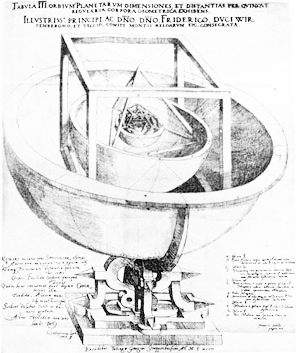Euclidean Geometry Primary geometric forms of Euclidean Geometry – circle, triangle, square.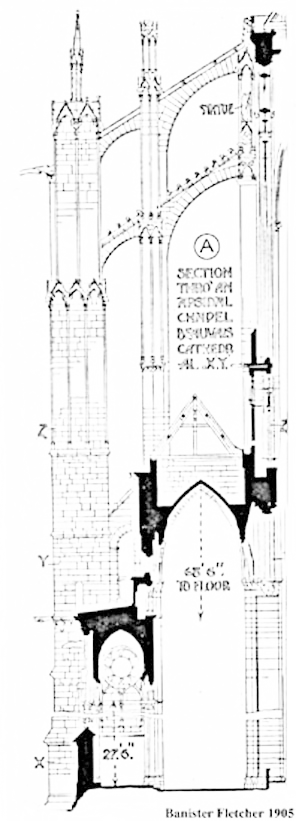Cross Sections of Beauvais Cathedral A comparison of the Cross sections of “Beauvais Cathedral” and “A Monument to Copernicus”.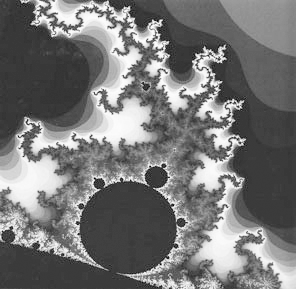Fractal Geometry Fractal Geometry: Being the most prominent aspect of Chaos, fractal geometry has the power to describe the complexity of nature. Two essential properties of fractal geometry are self-similarity and infinite details. Mandelbrot set provides a lucid example of this kind of geometry.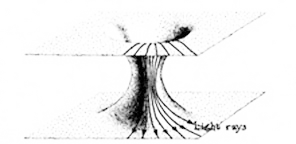Wormhole Kovalenko wormholeString A segment of string, which exists in 11th spatial dimension with 10⁻³³ cm in diameter.Nicolaus Copernicus Copernicus’s heliocentric model, with the Sun at the center of the universe, demonstrated that the observed motions of celestial objects can be explained without putting Earth at rest in the center of the universe. His work stimulated further scientific investigations, becoming a landmark in the history of science that is often referred to as the Copernican Revolution.Superstring Theory Superstring theory: The superstring theory proposes that subatomic particles are like elastic strings vibrating in space with the string size of a hundred bullion billionth of the diameter of an atomic nucleus, binding the nucleus together. The picture seen here consists of tubes swept out in space and time by loops representing particular particles. Where tubes join or separate, particles are colliding or being created.Albert Einstein Albert Einstein (1879-1955): German physicist who developed the theory of relativity, which provided a radical new outlook on space, time and gravitation, and for the first time proposed the equivalence of mass and energy.Clockwork Clockwork that symbolizes the predictability and three-dimensionality of Newtonian cosmology.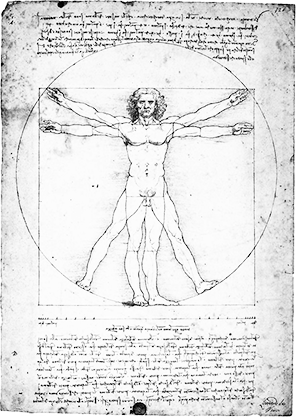Caesariano’s Vitruvian Man Caesariano’s Vitruvian Figure: Vitruvian human figure demonstrates the anthropocentric approach in Greek architecture. The perfect circle and sphere around the human figure are employed to provide a proof of the proportional perfection of human body. The use of such proof in turn, suggests the dominating power of Euclidean geometry in Greek architecture.Persistence of Memory The Persistence of Memory: Salvador Dali’s droopy clocks in The Persistence of Memory (1931) suggest the often peculiar kinship of time to space= a relation, some scientists contend, made up of 11 dimensions.Curved Space The curved space: according to Einstein’s General Theory of relativity, light beam will bend under the influence of gravity while passing by a celestial body. Based on “Fermat’s least-time principle” light beam will take the path requiring the least amount of time between two points. The fact that the light beam curves the presence of celestial body, then, logically suggests the shortest distance between two points could be a curved line. The only explanation of a curved line being the shortest path between two points is that the space itself is curved under gravitational pull of the celestial body.Quantum Particles The deformed spheres, which contain digital planetarium, 4-D theater and I-Werk theater, signify fundamental particles in Quantum Mechanics.
Super StringUsing curvy tubular strings, as here rendered by artist, to create functional spatial elements that contain digital planetarium, 4-D theater and I-Werk theater.